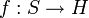# Reduced free group

This article defines a group property: a property that can be evaluated to true/false for any given group, invariant under isomorphism
View a complete list of group properties
VIEW RELATED: Group property implications | Group property non-implications |Group metaproperty satisfactions | Group metaproperty dissatisfactions | Group property satisfactions | Group property dissatisfactions

## Definition

No. Shorthand A group is termed a reduced free group if ... A group$G$ is termed a reduced free group if ...
1 Quotient of free it is isomorphic to the quotient of a free group by a verbal subgroup there is a free group$F$ and a verbal subgroup$V$ of$F$ such that$G \cong F/V$
2 Free in some subvariety it is a free algebra in some subvariety of the variety of groups. there is a subvariety$\mathcal{V}$ of the variety of groups such that$G$ is a free algebra in that subvariety. More explicitly, there is a generating set$S$ for$G$ such that for any$H \in \mathcal{V}$, any set map$f:S \to H$ extends uniquely to a group homomorphism from$G$ to$H$.
3 Free in own subvariety it is a free algebra in the subvariety of the variety of groups generated by itself.$G$ is a free algebra in the subvariety$\mathcal{V}(G)$ (i.e., the subvariety generated by$G$) in the variety of groups.

## Relation with other properties

### Stronger properties

Property Meaning Proof of implication Proof of strictness (reverse implication failure) Intermediate notions
Free group free in the variety of groups |FULL LIST, MORE INFO
Free abelian group free in the variety of abelian groups |FULL LIST, MORE INFO
Elementary abelian group trivial or abelian of prime exponent |FULL LIST, MORE INFO
Burnside group free in the variety of groups of exponent dividing$d$, for some pre-specified$d$ |FULL LIST, MORE INFO
Finite homocyclic group finite direct power of a finite cyclic group |FULL LIST, MORE INFO
Free class two group free in the variety of groups of nilpotency class two |FULL LIST, MORE INFO
Free metabelian group free in the variety of metabelian groups |FULL LIST, MORE INFO

### Weaker properties

Property Meaning Proof of implication Proof of strictness (reverse implication failure) Intermediate notions
Group in which every fully invariant subgroup is verbal every fully invariant subgroup is a verbal subgroup fully invariant implies verbal in reduced free simple groups give counterexamples |FULL LIST, MORE INFO